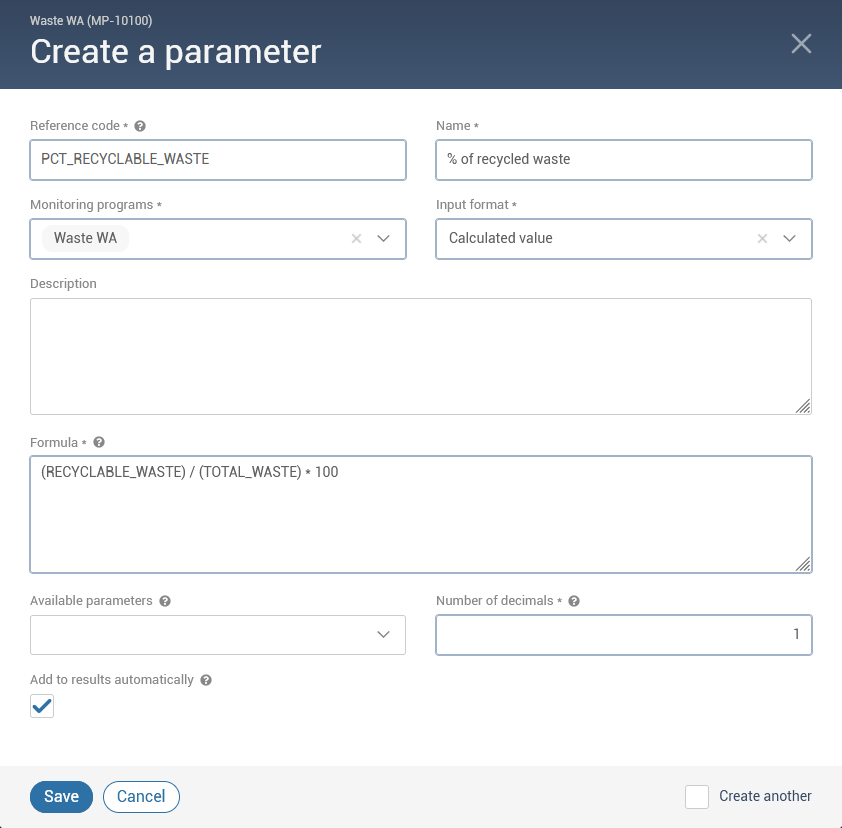# Create Calculated Parameters

Parameters are managed in the Parameters register. When adding a new parameter, select Calculated value as the Input format. Once this format is selected, four additional fields become available which allow you to customize your formula.

For example, you might want to know the Percentage of recyclable waste. However, the two parameters for which information is collected are the Total waste (kg) and the Recyclable waste (kg). The example below shows how to enter the formula to calculate the percentage of recyclable waste.Formula field
The Formula field is used to enter the formula to be calculated for your parameter. Accepted symbols, operators and variables are the followings:

• Mathematical operators: +, -, *, ^, % and /
• Parentheses: '(' and ')'
• Numbers
• Import code of available parameters

Available parameters field
Available parameters are listed in the Available parameters field. You can select parameters to automatically add them to the formula without having to type their reference code manually.

A parameter is available if:

• its input format is "Numeric" or "Numeric with symbol"
• it has at least one monitoring program in common with the new parameter
• it has a valid reference code (letters, numbers and underscore symbol characters only and a minimum of one letter)